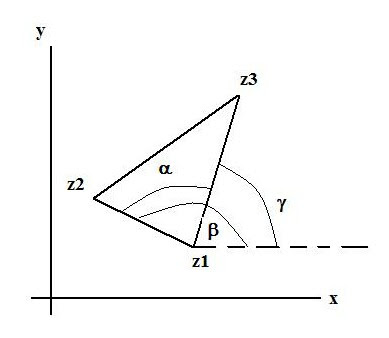## Monday, March 29, 2010

### Principal Value problemsSome problems on principal values for the ambitious reader:

Problems:

1. Find the principal value of (1 – i)^3

2. Find Arg(z) if z = 2i exp[-i(3pi/ 4)]

In general, the identity holds that: Arg(z1 z2) = Arg(z1) + Arg(z2)

Show it doesn’t hold if z1 = (-1 + i(3)^1/2) and z2 = -(3)^1/2 + i

Show an instance it does hold by identifying z1 and z2 that are appropriate and then showing the working.

3.Find the principal value for log(z) where z = (3)^1/2 –i

4. Find Arg(z) for:

a) z = 8 [exp i(7pi/ 3)]

b) z = exp(2) exp(i pi)

For the super -ambitious math whiz:

5. Let z1, z2 and z3 form the vertices of a triangle as indicated in the diagram. Show that:

alpha = arg{(z2 -z1)/ (z3 - z1)} = arg (z2 - z1) - arg(z3 - z1)

Hint: Any displacement vector in the complex plane: viz., (z - z0) can be formulated as a polar form:

(z - z0) = r(cos q + isin q)

say to measure the distance from z0 to z.